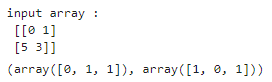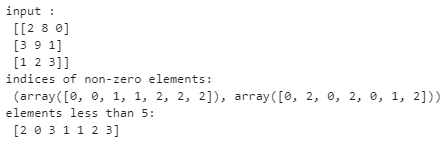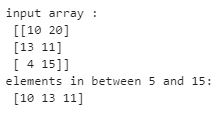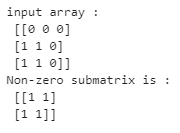# NumPy nonzero## Introduction to NumPy nonzero

A non-zero Function from the numpy module in python is used to find the indices of non-zero elements in a numpy array. The numpy.nonzero() function returns a tuple of arrays with indices of all the non-zero elements in the array. For the uninitiated, a numpy array is like a matrix of elements. In this article, we will look at the syntax of numpy.nonzero() function and then discuss a few examples based on it. To begin with, let’s first try to understand some of the possible uses of this function.

### Uses of numpy.nonzero() function

• The popular usage of the numpy nonzero function is to find the indices of elements of the array that satisfy a certain logical condition. For example, finding the indices of elements in the array which is greater or less than 1, even, etc.
• Another popular usage of this function is to find non-zero submatrices within a numpy matrix. For example, you have a large matrix with zeros and a few non-zero elements, and you wish to extract the non-zero sections of the matrix.

Don’t worry, even if you don’t understand much now. We will discuss this further in the examples section. But before we begin with examples, let’s discuss the syntax and arguments of this function.

Syntax and Parameters

The basic syntax for numpy.nonzero() function is as follows :

`numpy.nonzero(a)`

Argument: The function takes an array-like instance as an input

Return: It returns a tuple with indices of non-zero elements in the input array

Having discussed the syntax, let’s discuss a few examples based on it.

### Examples of NumPy nonzero

Given below are the examples of NumPy nonzero:

#### Example #1

Find the indices of nonzero elements in the given input array.

Code:

```#import numpy module import numpy as np #input a = np.array([[0,1],[5,3]]) print('input array :\n',a) #indices of non-zero elements np.nonzero(a)```

Output:In the above output, you will observe that the np.nonzero function returns a tuple that contains indices for each nonzero element in the array.  In this particular example, indices [0,1], [1,0], [1,1]. The first value of the tuple corresponds to the first index of the nonzero elements, i.e. (0,1,1), and the second value in the tuple corresponds to the second index of each non-zero element, i.e. (1,0,1). You may consider it as a transpose of the indices.

#### Example #2

Find the elements that are less than 5 from the given input array.

Code:

```#input a = np.array([[2,8,0],[3,9,1],[1,2,3]]) print("input :\n",a) #nonzero function with condition print('indices of non-zero elements:\n',np.nonzero(a #returning elements less than 5 print('elements less than 5:\n', a[np.nonzero(a```

Output:In this example, we have used a condition along with the np.nonzero function. The function returns the indices of all the elements in the array that meet the specified condition. Here, 1 is considered as True and 0 as False. For example, elements (2,0,3,1,1,2,3) satisfy the condition. Their respective indices are [0,0], [0,2], [1,0], [1,2], [2,0], [2,1] and [2,2].

#### Example #3

Find the elements that are more than 5 but less than 15 from the given input array.

Code:

```#input a = np.array([[10, 20], [13, 11], [4, 15]]) print('input array :\n',a) #nonzero function with combinatorial logic print('elements in between 5 and 15:\n',a[np.nonzero((a > 5) & (a ```

Output:This example is similar to the previous one, with just the exception that here we have used a combination of conditions. So, for example, you can use complex conditions joined together by logical operators like ‘&’(and) and ‘|’(or).

#### Example #4

Spot and extract the smallest submatrix with nonzero values from the given input matrix.

Code:

```#function using np.nonzero() def nonzero_submat(a): #non-zero indices x, y= np.nonzero(a) #the lower and upper bound of the non-zero indices in the array x_low_ind = x.min() x_high_ind = x.max() y_low_ind = y.min() y_high_ind = y.max() return a[x_low_ind:x_high_ind+1,y_low_ind:y_high_ind+1] #input a = np.array([[0, 0, 0], [1, 1, 0], [1, 1, 0]]) print('input array :\n',a) #calling function on input print("Non-zero submatrix is :\n", nonzero_submat(a))```

Output:You can get as creative as you want with a nonzero function. You can use it as a feeder function for your custom functions, as shown in this example. In this example, we tried to extract the smallest submatrix from the matrix. First, we have found indices of non-zero and then used those elements as upper and lower bound of the smallest possible submatrix.

### Conclusion

The nonzero function from numpy is a very popular function that is used to find the indices of nonzero elements or satisfy a specified condition in an array.

### Recommended Articles

This is a guide to NumPy nonzero. Here we discuss the Uses of numpy.nonzero() function along with the Examples, Syntax, and parameters. You may also have a look at the following articles to learn more –

2. Numpy.argsort()
3. NumPy Arrays
4. NumPy Linear Algebra

The post NumPy nonzero appeared first on EDUCBA.

This post first appeared on Best Online Training & Video Courses | EduCBA, please read the originial post: here